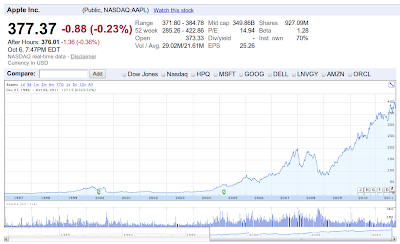## 2011年10月7日 星期五

jobs 是不是英雄? 在我年紀小的時候當然是，但現在我可不確定了。3 塊多的股價大概是在 1997 年，也差不多是我大學時代的事。與我開始買股票的經驗相仿，不過 i蘋果一書，卻說 1997 左右，它的股價在 16 塊附近，這我就不懂了。不知道是誰對。

== 後記 ==

## 2011年10月1日 星期六

### 另一種解鋼筋切割組合問題(無界背包問題)的方法(改自 thinker 所提觀念)

`#!/usr/bin/env python# -*- coding: utf8 -*-""" cut(10, [7, 5, 3, 2]) = cut(3, [7, 5, 3, 2]) + cut(10, [5, 3, 2])"""def _plus1(a):    a += 1    return adef _cache(my_function):    CACHE = {}    def inner_function(*args):        key = str(args[:])        if not CACHE.get(key, None):            CACHE[key] = my_function(*args)        return CACHE[key]    return inner_function@_cachedef cut(total, sizes):    propers = tuple([sz for sz in sizes if sz <= total])    if len(propers) == 1:        return [[0,]*(len(sizes)-len(propers))+[total/propers,],]    elif not propers:        if total < 0:            return []        else:            return [[0,]*len(sizes),]    result1 = [_plus1(a) for a in cut(total-sizes, sizes)]    result2 = [[0, ]+a for a in cut(total, sizes[1:])]    return result1 + result2if __name__ == '__main__':    from time import time    bar = 10    sizes = [7, 5, 3, 2]    t0 = time()    answer = cut(bar, sizes)    print time() - t0    print 'count: %s'%len(answer)    #for a in answer:    #    print a, sum([j*sizes[i] for i, j in enumerate(a)])`

### 2011 被動投資回顧

2009: 3%
2010: 3.6%
2011: 5%(以 17% 扣抵稅率試算)